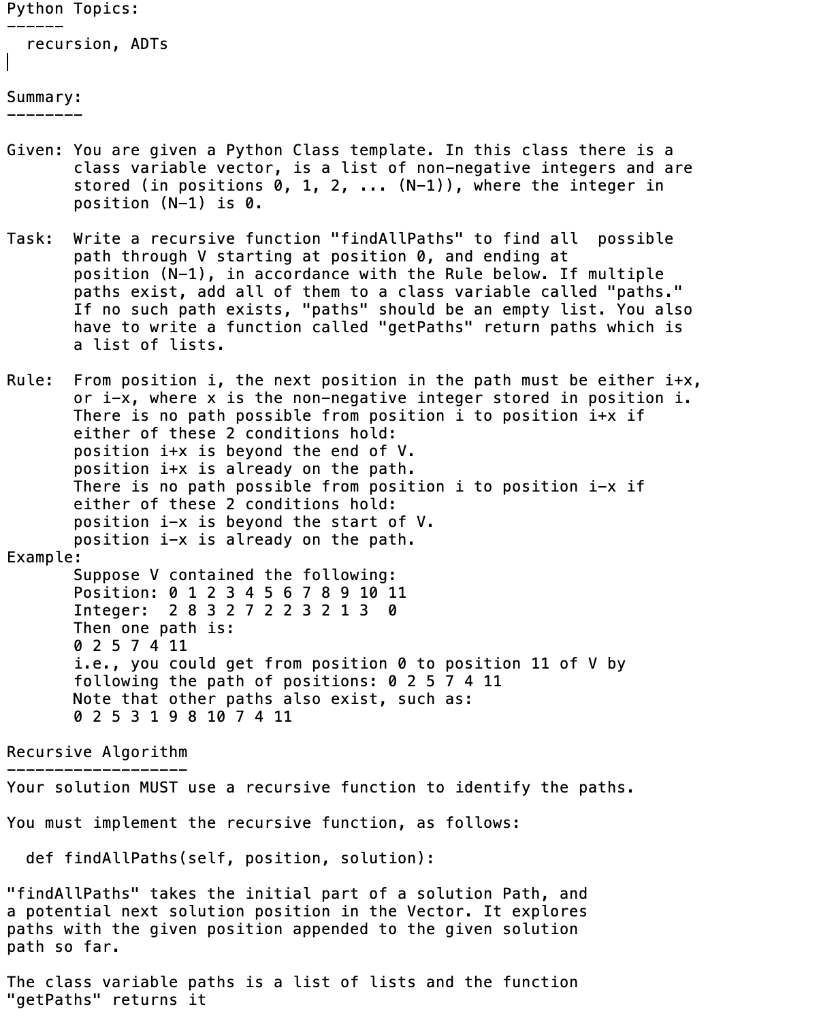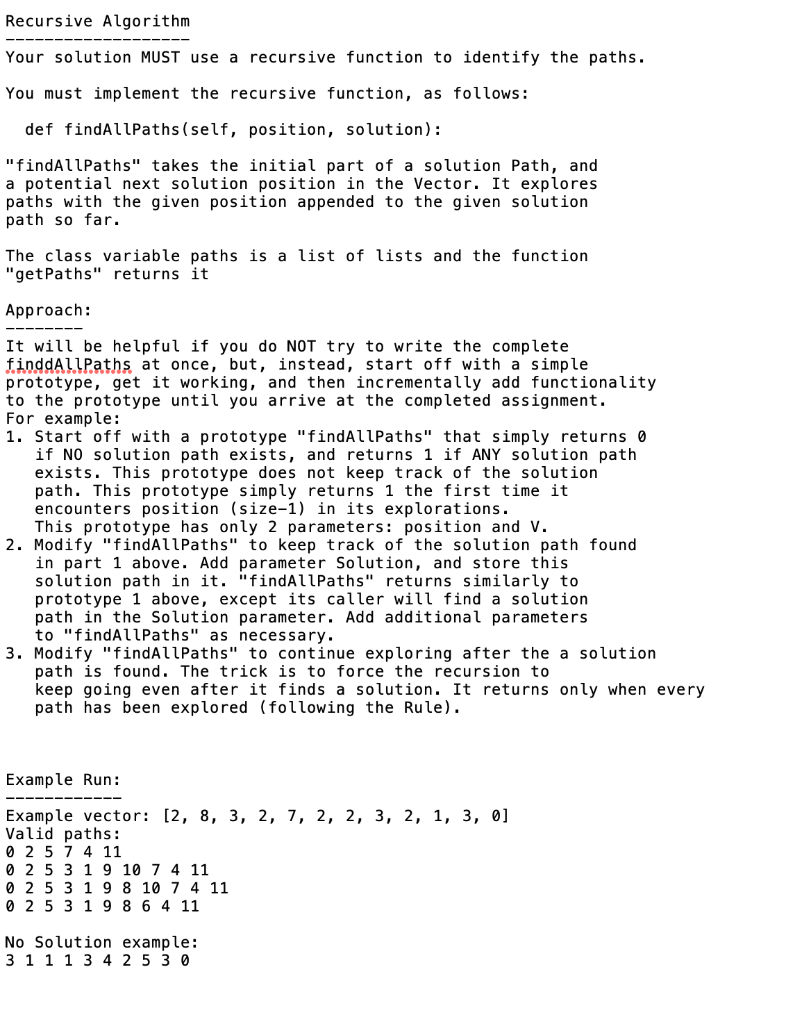# (Solved) : Templatepy Class Pathfinder Def Init Self Vector Initialize Pathfinder Object Selfvector V Q42769949 . . .#template.py class Pathfinder():    def __init__(self, vector):        # Initialize thePathfinder object        self.vector =vector        self.paths = []     def findAllPaths(self, position,solution):        # Recursively explorethe possible paths and store valid paths        # This method will notbe tested, so you can modify the parameters as needed        pass     def getPaths(self):        # Return the list ofviable paths, or [None] if there are no solutions        pass # The Pathfinder class is initialized with a list of integers,the target of which is the position with value 0.# The findAllPaths method recursively explores the list.# The getPaths method returns the list of viable paths, or [None]if there are no solutions # vectortester.py import randomimport stringimport re#Module Importsimport sysfrom importlib import import_module def generate(size):    arr = [None] * size     arr[size-1] = 0      for i in range(0, size-2):        arr[i] =random.randint(1, size)     return arr def pathTest(vector, path):    def pathTest_(vector, path, position,location):        if(location ==len(path)-1):           if(position == len(vector) -1 ):               return True           else:               return False        elif location >=len(vector) or location < 0:           return False        else:           return pathTest_(vector, path, position + vector[path[location]],location +1 ) or pathTest_(vector, path, position -vector[path[location]], location +1 )     return pathTest_(vector, path, 0,0) def Test(lib, seed=0, size=10, verbose=False):    known = [        ([2, 8, 3, 2, 7, 2, 2,3, 2, 1, 3, 0], 4), # Many solutions, handle it        ([2,3,1,1,0], 2), #Simple infinite loops        ([3, 1, 1, 1, 3, 4, 2,5, 3, 0], 0), # Zero solutions       ([4,3,1,2,3,5,4,2,2,1,1,0], 5), # Complicated loops        ([4,4,1,2,3,1,8,2,0],0)# Infinite loops, zero solutions    ]    for (vector, solutions) in known:              finder =lib.Pathfinder(vector)        count = 0        if verbose:           print(f”Vector: {vector}”)        for path infinder.getPaths():           if pathTest(vector, path):               count+= 1               if verbose:                   print(“Valid soltion: “, end=””)                   print(path)           else:               if verbose:                   print(“Error: Invalid path returned”)               return False        if count !=solutions:           if verbose:               print(“Error: Incorrect number of solutions”)           return False        if verbose:           print()         yield True      if __name__ == “__main__”:      if len(sys.argv) < 2:        print(“Include at leasta library name as an argument.”)        exit()    name = sys.argv    if name.endswith(“.py”):        name = name[:-3]    print(f”Testing module {name}”)   module=import_module(name,package=__name__)    score=0    for i in Test(module,seed=123456, size=20,verbose=True):        if i:           score+=1        else:           break     print(f”Test result: {score}/5″)

Recursive Algorithm Your solution MUST use a recursive function to identify the paths. You must implement the recursive function, as follows: def findAllPaths (self, position, solution): “findAllPaths” takes the initial part of a solution Path, and a potential next solution position in the Vector. It explores paths with the given position appended to the given solution path so far. The class variable paths is a list of lists and the function “getPaths” returns it Approach: It will be helpful if you do NOT try to write the complete finddAllPaths, at once, but, instead, start off with a simple prototype, get it working, and then incrementally add functionality to the prototype until you arrive at the completed assignment. For example: 1. Start off with a prototype “findAllPaths” that simply returns 0 if NO solution path exists, and returns 1 if ANY solution path exists. This prototype does not keep track of the solution path. This prototype simply returns 1 the first time it encounters position (size-1) in its explorations. This prototype has only 2 parameters: position and V. 2. Modify “findAllPaths” to keep track of the solution path found in part 1 above. Add parameter Solution, and store this solution path in it. “findAllPaths” returns similarly to prototype 1 above, except its caller will find a solution path in the solution parameter. Add additional parameters to “findAllPaths” as necessary. 3. Modify “findAllPaths” to continue exploring after the a solution path is found. The trick is to force the recursion to keep going even after it finds a solution. It returns only when every path has been explored (following the Rule). Example Run: Example vector: [2, 8, 3, 2, 7, 2, 2, 3, 2, 1, 3, 0] Valid paths: 0 2 5 7 4 11 0 2 5 3 1 9 10 7 4 11 0 2 5 3 1 9 8 10 7 4 11 0 2 5 3 1 9 8 6 4 11 No Solution example: 3 1 1 1 3 4 2 5 30

Answer to #template.py class Pathfinder(): def __init__(self, vector): # Initialize the Pathfinder object self.vector = vector sel…

We are the best freelance writing portal. Looking for online writing, editing or proofreading jobs? We have plenty of writing assignments to handle.